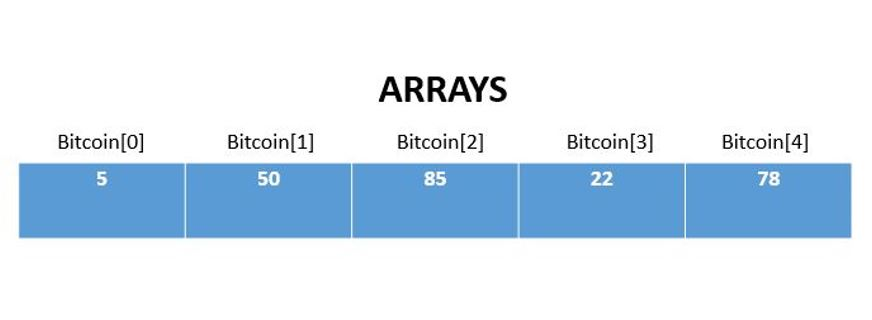# Arrays in JAVAAn array is a collection of variables which are of similar type. Arrays can be accessed with their indexes.

Arrays can be used to store numbers, list of values and other variables.

Consider the following example below :

int[ ] Bitcoin = new int;

Here, we are defining an array Bitcoin of integer datatype consisting of 5 Bitcoins.

We can reference each element in the array with an index, say the elements of the array are {5,50,85,22,78}. We can access the 2nd element by using Bitcoin. Note indexing in Java starts at 0.

Let us look into a program to access arrays by referencing:

public class Cryptocurrency {

public static void main(String[] args) {

String[ ] Blockchain = { “Amsterdam”, “Berlin”, “Capetown”, “Delhi”};

System.out.println(Blockchain);

}

}

Program to calculate length of an array.

public class Coins {

public static void main(String[] args) {

int[ ] Bitcoin = new int;

System.out.println(Bitcoin.length);

}

}

Program to find sum of an array.

public class Coins {

public static void main(String[] args) {

int [ ] Bitcoin = {5,50,85,22,78};

int sum=0;

for(int k=0; k<Bitcoin.length; k++) {

sum += Bitcoin[k];

}

System.out.println(sum);

}

}

In this code, the variable sum stores all the variables by adding all the elements of array. Initially the sum is assigned a value of zero. A for loop traverses through the array list because of the expression k<Bitcoin.length, that is the number of elements will be equivalent to a value less than length of the array. Remember, the indexing starts at  for arrays.

Printing values of an Array.

public class Coins {

public static void main(String[] args) {

int[ ] values = {5,50,85,22,78};

for (int P: values) {

System.out.println(P);

}

}

}

2D and 3D Arrays

Accessing 2D Array

public class Coins {

public static void main(String[] args) {

int[ ][ ] Bitpart = { {77, 55, 34}, {41, 54, 63} };

int j = Bitpart;

System.out.println(j);

}

}

Accessing 3D Array

public class Coins {

public static void main(String[] args) {

int[ ][ ] Bitpart = { {17, 21, 32}, {41,70,44}, {51, 62, 75} };

Bitpart = 32;

int m = Bitpart;

System.out.println(m);

}

}

Similarly, N dimensional arrays can also be accessed.

```Source:1)THE Java™ Programming Language, Fourth Edition

By Ken Arnold, James Gosling, David Holmes

2) SoloLearn Online Platform.```